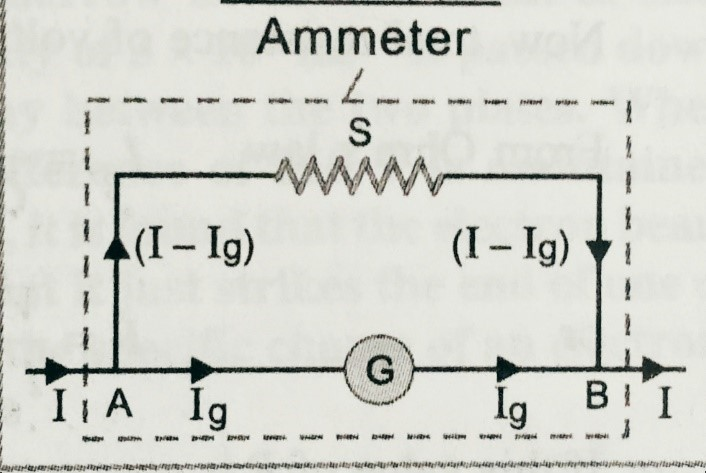Categories

Conversion of galvanometer into ammeter

In this topic we will discuss about the ‘ Conversion of galvanometer into ammeter ‘ . What is ammeter? and how can we convert a voltmeter into ammeter?

Before to read this topic ‘ Conversion of galvanometer into ammeter ‘  students must know about the galvanometer and shunt .

Ammeter –

It is a low resistance galvanometer used to measure the electric current in a circuit.

Let, I and IG are the total current passes through the circuit and the galvanometer respectively. G , and S are the resistances of the galvanometer and shunt respectively.To know the syllabus of class 12th board  examinations ( CBSE ) click here-

As shown in figure,  potential across S is VS = (I- IG ) S

Potential across  G is  VG = IG G

Since both are in parallel combination , hence  is  VS = VG

So, ( I-IG) S =  IG G

S=  IG G/( I-IG) ……………………….( i)

Using this  equation we can find the value of shunt to get the ammeter of our desired value.

Resistance of the Ammeter ;  Let R is the resistance of the ammeter.

Then we can write ;  1/g  + 1/s = 1/ R

R = S+G/SG ;

For ideal ammeter value of R should be  zero.

After studding this topic students can visit the next topic which is conversion of galvanometer into voltmeter. To get the next topic click on the link given below.